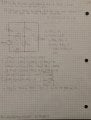# Mesh Current - Difficult

#### Godsninja

Joined Apr 30, 2016
16
Hi again! I've refreshed myself on mesh-current, but this 1 problem (of 2) has stumped me. It's doesn't look overly difficult (topic name is to keep consistency) but my answers are wrong. I've taken a picture of the question, circuit, and my solution. The question states to find the branch currents from ia through ie

The second part of the question (b) can be ignored in this topic.

At first I was a little unsure about i3. I thought it was 4.3id - i2. After fixing that mistake, my solution and texts solutions are still different. The rest is evident. I don't know what else to say because I've looked over this numerous times and I can't find any flaws in my method.

The question is from Nilsson & Riedels, Electric Circuits 9th edition.

Thanks.#### WBahn

Joined Mar 31, 2012
25,751
You claim that:

$$i_3 \; = \; 4.3 i_d$$

Are you sure you agree with this?

If so, then why isn't id = i2?

I'm very happy to see you making a real effort to use units, but you are doing two things wrong.

First, you have things like

$$i_3 \; = \; 4.3 i_d \; A$$

You have units of current squared, because id has units of current.

And what if id happens to be 10 mA. Now you have 43 mA².

Next, you start dropping your units partway through your work. You dropped the Ω and so you have an equation in which you have a current plus a current equals a voltage.

Then you further drop the units entirely when you solve for i1 and i2 and have them as pure numbers instead of currents.

Don't worry -- it takes time, patience, and attention to detail.

#### BlackMelon

Joined Mar 19, 2015
84
i3 = 4.3id..... or i3-i2 = 4.3idBe careful with the KVL method.

#### Godsninja

Joined Apr 30, 2016
16
You are right, I could have tried harder. And thank you BlackMelon, that makes perfect sense.

I would really like to upload a photo of the final solution, some of my best work (when it comes to using units properly), but the website won't let me, giving me an error. I tried multiple times, but it won't let me.

Here's an un-editted un-scaled version directly from my drive though: https://photos.app.goo.gl/WJVH0XCDUbA3yHM72

Also, looks like the person who did the question for the publisher got ic and ie reversed.

Thanks again!

#### WBahn

Joined Mar 31, 2012
25,751
You are well on the way to doing exceptional work. Most of the next set of comments I was going to make about your prior attempt you have dealt with in the latest one.

You have now labeled your two mesh equations in a meaningful way that shows that the second one is a supermesh equation. This is extremely helpful to someone trying to understand your work.

Another problem your earlier work had is that the work to the right of the diagram involved results from work done below the diagram. This makes it very hard to follow. Your work should flow in a continuous direction down the page if at all possible.

About the only other recommendation I have at this point is to first get your equations done but don't manipulate them. So I would have put the first equation that you have to the right of the diagram as the first equation underneath it. Then the two mesh equations. Then draw a line under these (basically where the center hole in the page is). Everything above this line is the EE stuff. Everything below this line is just math. Before you proceed, review the three equations that you have above the line and be sure that you are satisfied that they are correct (this step would likely have caught your mistake). This is critical, because no amount of math can catch a mistake that you make in setting up the EE equations -- so you want those equations to be as obvious as you can make them. I think your basic problem was that you were trying to do two things at once to the right of your diagram -- apply the EE concepts AND do a bunch of math manipulations. Humans aren't good at multi-tasking, no matter what we claim. So focus on JUST the EE stuff. Check it. Then proceed. Once you are below the line, you are ONLY doing algebra and can focus on that, thus reducing the chances of letting a math error slip by because you are trying to do EE stuff at the same time.

The order I would have presented the EE equations are:

Mesh 1
Mesh 2,3
Constraint 2,3

The equation that relates currents i2 and i3 is a constraint equation that is needed because you used a supermesh equation involving i2 and i3.

•Godsninja